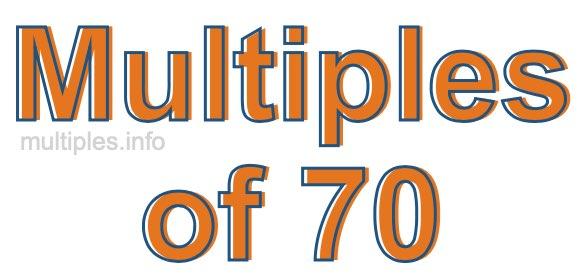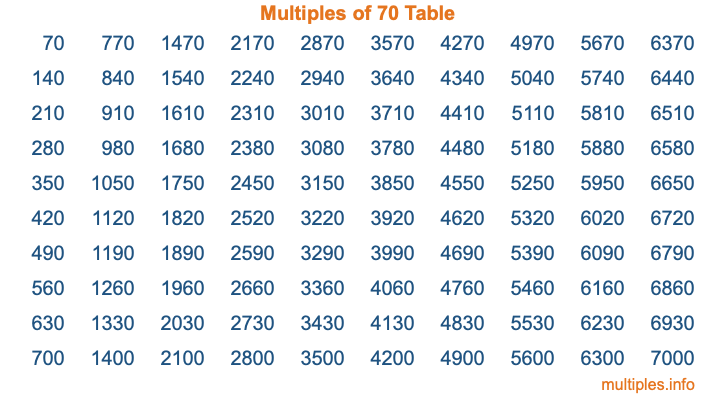Multiples of 70Welcome to the Multiples of 70 page. Here we will first teach you everything you will ever need to know about the multiples of 70, and then give you a study guide summary of everything we taught you to make sure you remember it all. Use this page to look up facts and learn information about the multiples of 70. This page will make you a multiples of seventy expert!

Definition of Multiples of 70
Multiples of 70 are all the numbers that when divided by 70 equal an integer. Each of the multiples of 70 are called a multiple. A multiple of 70 is created by multiplying 70 by an integer.

Therefore, to create a list of multiples of 70, you start with 1 multiplied by 70, then 2 multiplied by 70, then 3 multiplied by 70, and so on for as long as you want. Thus, the list of the first five multiples of 70 is 70, 140, 210, 280, and 350. To see a larger list of multiples of 70, see the printable image of Multiples of 70 further down on this page. We also have a category where you can choose any nth multiple of 70.

Multiples of 70 Checker
The Multiples of 70 Checker below checks to see if any number of your choice is a multiple of 70. In other words, it checks to see if there is any number (integer) that when multiplied by 70 will equal your number. To do that, we divide your number by 70. If the the quotient is an integer, then your number is a multiple of 70.

Is  a multiple of 70?

Least Common Multiple of 70 and ...
A Least Common Multiple (LCM) is the lowest multiple that two or more numbers have in common. This is also called the smallest common multiple or lowest common multiple and is useful to know when you are adding our subtracting fractions. Enter one or more numbers below (70 is already entered) to find the LCM.

Check out our LCM Calculator if you need more details about the Least Common Multiple or if you need the LCM for different numbers for adding and subtraction fractions.

nth Multiple of 70
As we stated above, 70 is the first multiple of 70, 140 is the second multiple of 70, 210 is the third multiple of 70, and so on. Enter a number below to find the nth multiple of 70.

th multiple of 70

Multiples of 70 vs Factors of 70
70 is a multiple of 70 and a factor of 70, but that is where the similarities end. All postive multiples of 70 are 70 or greater than 70. All positive factors of 70 are 70 or less than 70.

Below is the beginning list of multiples of 70 and the factors of 70 so you can compare:

Multiples of 70: 70, 140, 210, 280, 350, etc.

Factors of 70: 1, 2, 5, 7, 10, 14, 35, 70

As you can see, the multiples of 70 are all the numbers that you can divide by 70 to get a whole number. The factors of 70, on the other hand, are all the whole numbers that you can multiply by another whole number to get 70.

It's also interesting to note that if a number (x) is a factor of 70, then 70 will also be a multiple of that number (x).

Multiples of 70 vs Divisors of 70
The divisors of 70 are all the integers that 70 can be divided by evenly. Below is a list of the divisors of 70.

Divisors of 70: 1, 2, 5, 7, 10, 14, 35, 70

The interesting thing to note here is that if you take any multiple of 70 and divide it by a divisor of 70, you will see that the quotient is an integer.

Multiples of 70 Table
Below is an image of the first 100 multiples of 70 in a table. The table is in chronological order, column by column. The first column has the first ten multiples of 70, the second column has the next ten multiples of 70, and so on.The Multiples of 70 Table is also referred to as the 70 Times Table or Times Table of 70. You are welcome to print out our table for your studies.

Negative Multiples of 70
Although not often discussed or needed in math, it is worth mentioning that you can make a list of negative multiples of 70 by multiplying 70 by -1, then by -2, then by -3, and so on, to get the following list of negative multiples of 70:

-70, -140, -210, -280, -350, etc.

Multiples of 70 Summary
Below is a summary of important Multiples of 70 facts that we have discussed on this page. To retain the knowledge on this page, we recommend that you read through the summary and explain to yourself or a study partner why they hold true.

There are an infinite number of multiples of 70.

A multiple of 70 divided by 70 will equal a whole number.

70 divided by a factor of 70 equals a divisor of 70.

The nth multiple of 70 is n times 70.

The largest factor of 70 is equal to the first positive multiple of 70.

70 is a multiple of every factor of 70.

70 is a multiple of 70.

A multiple of 70 divided by a divisor of 70 equals an integer.

70 divided by a divisor of 70 equals a factor of 70.

Any integer times 70 will equal a multiple of 70.

Multiples of a Number
Here you can get the multiples of another number, all with the same attention to detail as we did for multiples of 70 on this page.

Multiples of
Multiples of 71
Did you find our page about multiples of seventy educational? Do you want more knowledge? Check out the multiples of the next number on our list!